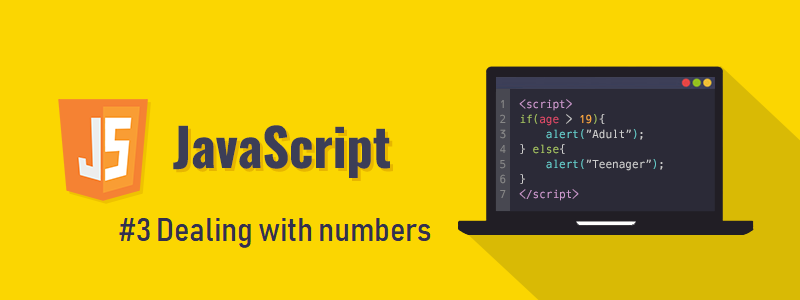## CodeNewbie Community 🌱

Rawan Amr Abdulsattar

Posted on • Originally published at codewithrona.blogspot.com

# Javascript : Dealing with numbersIn Javascript , you can do operations on numbers to help you do calculations and make things easier.

# Basic operations on numbers

• Addition : using " + " operator
``````var sum ;
sum = 10 + 5 ;
console.log(sum); // output : 15``````
• Subtraction : using " - " operator
``````var difference ;
difference = 22 - 10 ;
console.log(difference); // output : 12``````
• Division : using " / " operator
``````var quotient ;
quotient = 50 / 5 ;
console.log(quotient); // output : 10``````
• Multiplication : using " * " operator
``````var product ;
product = 7 * 2;
console.log(product); // output : 14``````
• Getting remainder of division : using " % " operator
``````var remainder ;
remainder = 10 % 4;
console.log(remainder); // output : 2``````
• Raising a number to a power : using the " ** " operator
``````var num = 3;
squaredNum = num ** 2;
console.log(squaredNum) // output : 9
``````

# Augmented operations on numbers

Sometimes , you want to update a variable storing a numeric value by doing any of the operations (like : adding to it , subtraction from it , dividing or multiplying it by something).class="javascript">But you don't have to use the long form like the following one.
``````var num = 2 ;
// adding 3 to the value of num and assigning this value (5) to the variable num
num = num + 3 ;
``````
You can shorten it to be as the following example
``````var num = 2;
// adding 3 to the value of num and assigning this value (5) to the variable num
num += 3 ;
``````

In the previous example , we used the plus equals operator " += "
which does the same thing as the code example before it.

This can be used in the operations (+, -, , /).

``````var num = 20 ;
// examples of augmented operations that can be done
num += 2 ;
num -= 3 ;
num *= 5 ;
num /= 11 ;
``````

You can replace the value you use to update your variable by another variable as in the following example

``````var salary = 1500;
var bonus = 250;
console.log(salary); // output : 1500
salary += bonus;
console.log(salary); // output : 1750
``````
Another example

``````var maxTemperature = 32;
var minTemperature = 24;
var averageTemperature =  ( maxTemperature + minTemperature ) / 2 ;
console.log(averageTemperature); // output : 28
``````

# Incrementing and decrementing by one

Sometimes you want to update variables by incrementing or decrementing them by one, instead of using (num += 1 or num -= 1) , you can use a shorter way using " ++ " and " -- " operators./p>

``````var num = 6;
num++; // increases it by one to be 7
num--;  // decreases it by one to be 6 again
``````

# Order of operations

If you have a more complex calculation you have to know what is calculated first , the order of operations that the computer follows is the same as the one you studied in your math's classes, which is PEDMAS (from left to right) :

• Parenthesis (Brackets)
• Exponents ( powers )
• Division and Multiplication

Some people make it funnier and easier to remember by saying it like : " please excuse my dear aunt Sally ! "
Examplein ( 2 * (4 + 6) * 2 / 5 - 1) :
• first ( 4 + 6 )  is calculated to be 10 ,
• then it's raised to the second power to be 100 ,
• then it's multiplied by 2 to be 200 ,
• after that, it's divided by 5 to be 40 ,
• and finally, 1 is subtracted from it to be 39.